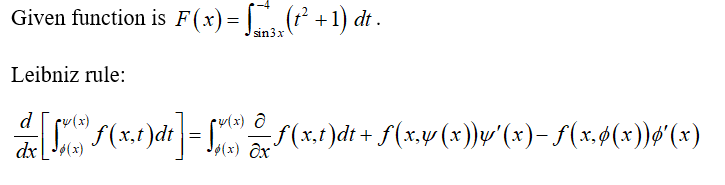# Find F'(x), where F(x)= int. of ((t^2)+1)dt, from sin(3x) to x=-4. Use the 1st rule of the fundamental theorem of calulus and leibniz rule.

Question
1 views

Find F'(x), where F(x)= int. of ((t^2)+1)dt, from sin(3x) to x=-4. Use the 1st rule of the fundamental theorem of calulus and leibniz rule.

check_circle

star
star
star
star
star
1 Rating
Step 1...

### Want to see the full answer?

See Solution

#### Want to see this answer and more?

Solutions are written by subject experts who are available 24/7. Questions are typically answered within 1 hour.*

See Solution
*Response times may vary by subject and question.
Tagged in